# Depth of Field, Part II: The Math

18ShareIn Part I we talked, in a fair amount of detail, about what DOF is and how it is determined. If you are happy with what you learned in Part I and do not feel the need to know the math behind all of the different factors in DOF, then you are free to go out and start confidently manipulating DOF while taking photos. However, if you are having difficulty pressing the “I believe” button and want to see the “concept” or “theory” of DOF become a cold, hard fact with mathematics, then keep on reading.

Circle of Confusion

Were you confused by the first foray in to the topic of Circle of Confusion (COC) in Part I? Well, the COC is a confusing, yet important part of how DOF is calculated. As we mentioned in Part I, there are three things that factor into the COC:

1. Viewing distance
2. Enlargement
3. Visual acuity

Here, in Part II, we will dive more deeply into the elements of COC so we can enter the numbers into a formula and then use this to figure out hyperfocal distance and then use that figure to calculate DOF mathematically.

Viewing distance is measured in centimeters for the purposes of our equation.

Enlargement is measured as a factor of magnification. In the example below, an 8 x 10" print is 7x larger than a 24 x 36mm sensor or frame of 35mm film.

Visual acuity is measured by line pairs per millimeter (lp/mm). At your optician’s office, your eyes are measured and the acuity is assigned a number; 20/20 being “normal” vision. This means that at 20' you can see clearly what a “normal” eye can see clearly at 20'. If you need to be at 20' to see what a normal eye sees clearly at 40', you have 20/40 vision. For the purposes of COC, a line pair comprises alternating black and white lines of equal size. You may have seen these lines on certain eye charts. Acuity is measured by determining how many line pairs a person can see at a designated distance. “Normal” vision is measured at 5 lp/mm.

Now let’s apply the COC factors into a simplified equation to mathematically resolve COC so we can use it in our DOF calculations.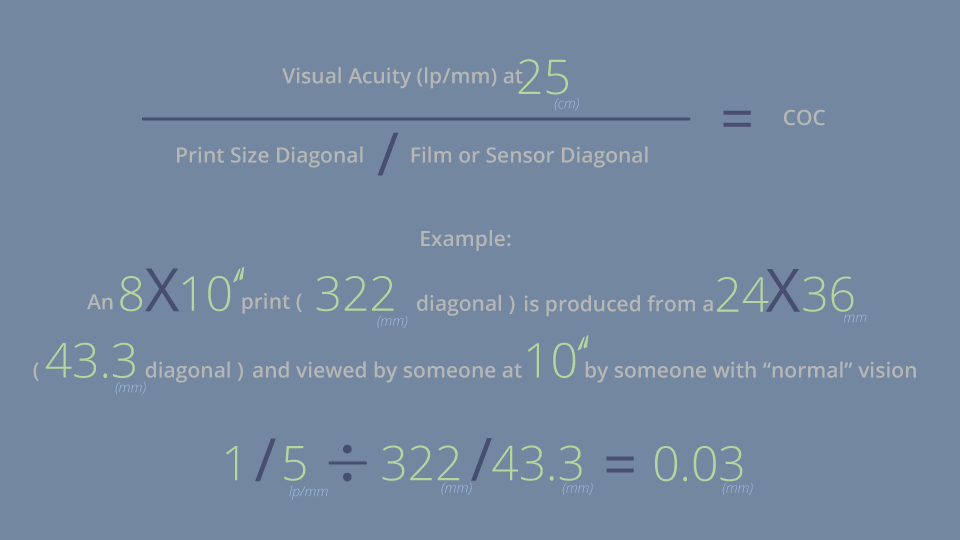The math behind COC

This COC value represents the maximum blur spot diameter, measured at the image plane, which looks to be in focus. A spot with a diameter smaller than this COC value will appear as a point of light and, therefore, in focus in the image. Spots with a greater diameter will appear blurry to the observer.

For simplicity, camera and lens manufacturers use a standard COC. The standard value varies between manufacturers, but it is generally around 0.03mm for full-frame cameras. The example above illustrates the variables used to formulate the COC.

Non-Symmetry of DOF

Are you ready for another wrinkle in your DOF world? DOF is not symmetrical. This means that the area of acceptable focus does not have the same linear distance before and after the focal plane. This is because the light from closer objects converges at a greater distance aft of the image plane than the distance that the light from farther objects converges prior to the image plane.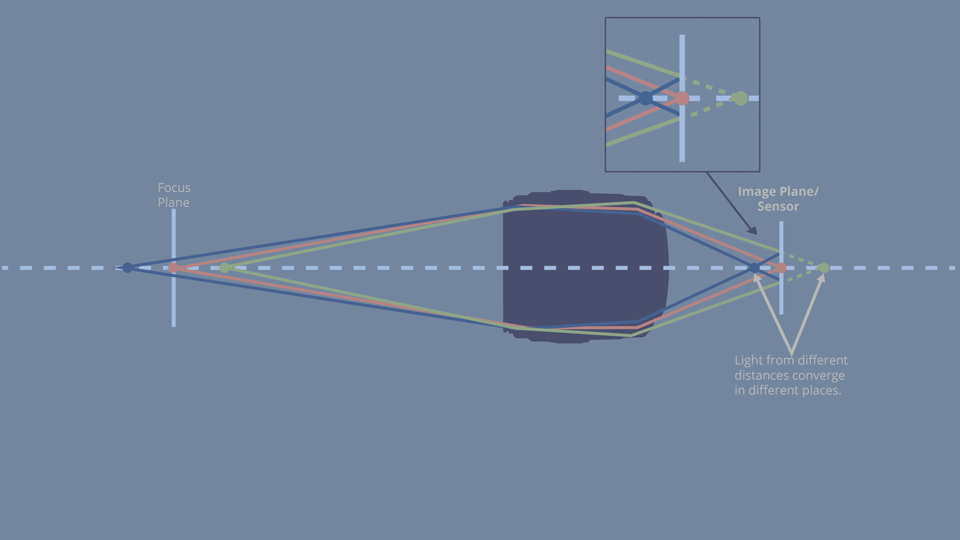Three equidistant objects. Once the light passes through the lens, the symmetry is shifted.

At relatively close distances, the DOF is nearly symmetrical, with about half of the focus area existing before the focus plane and half appearing after. The farther the focal plane moves from the image plane, the larger the shift in symmetry favoring the area beyond the focal plane. Eventually, the lens focuses at the infinity point and the DOF is at its maximum dissymmetry, with the vast majority of the focused area being beyond the plane of focus to infinity. This distance is known as the “hyperfocal distance” and leads us to our next section.

Hyperfocal Distance

Hyperfocal distance is defined as the distance, when the lens is focused at infinity, where objects from half of this distance to infinity will be in focus for a particular lens. Alternatively, hyperfocal distance may refer to the closest distance that a lens can be focused for a given aperture while objects at a distance (infinity) will remain sharp. The hyperfocal distance is variable and a function of the aperture, focal length, and aforementioned COC.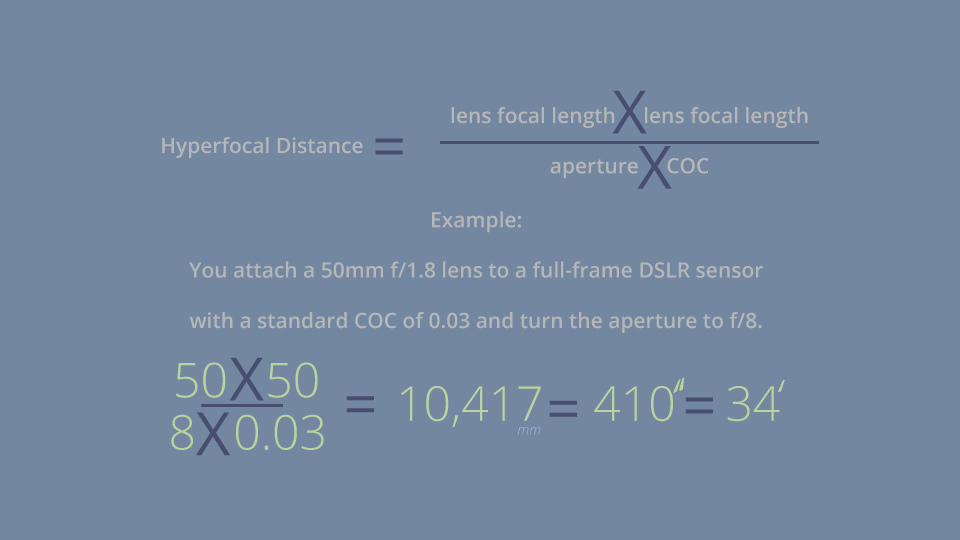The math behind hyperfocal distance

34' is the hyperfocal distance. If the lens is set at f/8 and focused at 34' (or at the infinity mark if it appears before 34'), everything from 17' to infinity should be in focus.

Remember that your solution will be presented in millimeters, as your lens focal length is most likely measured in millimeters. Using that formula, you will see that the smaller you make the lens aperture, the closer to the lens the hyperfocal distance becomes.

Back in the old days, lenses used to have hyperfocal distance markings on the lens barrels and/or near the focus rings. This is rarely seen in today’s autofocus world, but there are many smartphone applications and websites that will crunch the numbers for you so that you can determine your lens’s hyperfocal distance for a given aperture. I will discuss these calculators later.

The practical application of hyperfocal distance is that, in the above example, you can set your 50mm f/1.8 lens to f/8 and turn your focus dial (if marked) to 34' or ∞ and everything in your image from 17' to the horizon and beyond should be in acceptable focus.

Why is this important? Hyperfocal distance also featured in—you guessed it—the calculations used to compute DOF.

Calculating DOF

It is now time, finally, to calculate DOF. In review, the four factors that determine DOF are:

1. Circle of confusion (COC)
2. Aperture of the lens
3. Lens focal length
4. Focus distance (distance between lens and subject)

Above, we used the standard COC and then added the lens focal length and aperture to calculate the hyperfocal distance. Now, we can add the fourth factor, subject-to-lens distance, into an equation to figure out our DOF. As we now know, DOF is a linear range before and aft of the focal plane, and we also know that the DOF range is not symmetrical on each side of the focal plane.

Our next voyage into the mathematical realm is to figure the DOF near point (remember we are working in millimeters, so don’t forget to convert):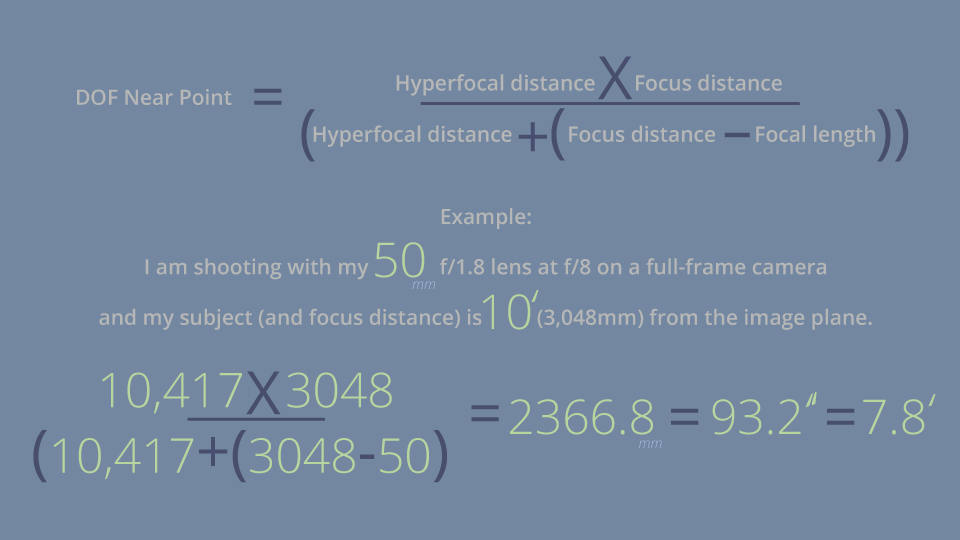The DOF Near Point is at 7.8'.

Ready to calculate the DOF Far Point now? Here we go: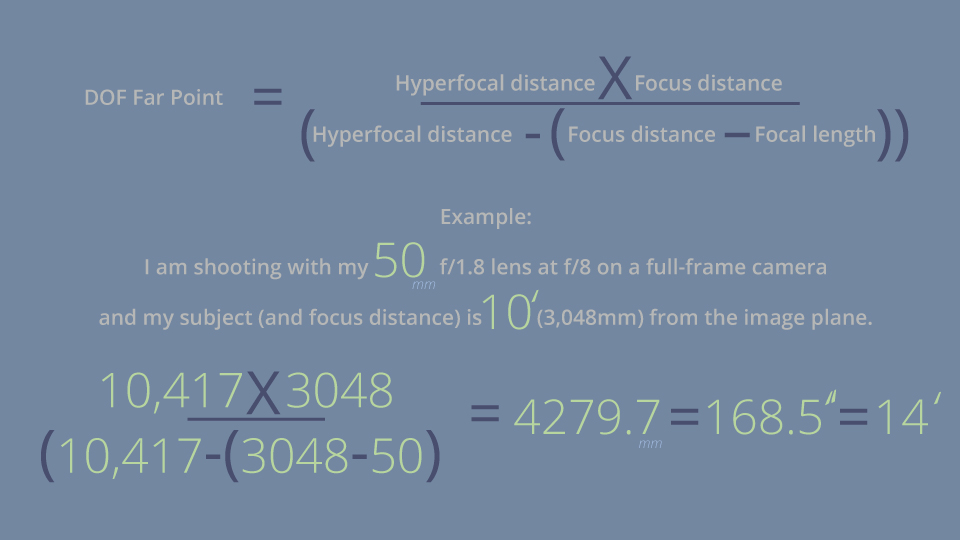The DOF Far Point is at 14.0'.

So, we know our camera is focused at 10' and we just crunched some numbers to show us that with a 50mm lens set to f/8, everything between 7.8' and 14.0' will be in acceptable focus. As you can see, the further range of DOF is greater than the near range.

Now, we will calculate the DOF:The DOF, the range of acceptable focus for the 50mm f/1.8 lens mounted on a full-frame camera, set to f/8, and focused at 10' is: 6.2'.

Last thing to mention: you might see or hear discussion of DOF being measured in “stops.” This is a misnomer. As you can see from the formulae and solutions, DOF is a distance, not a value for exposure. When someone mentions adding or subtracting a “stop” of DOF, they are likely referring to altering the DOF by changing aperture and, therefore, changing the DOF, but DOF is a linear measurement, not a value for exposure or light.

DOF Calculators

If you want to calculate your camera-and-lens combination’s DOF out in the field, there are a multitude of websites and smartphone applications that help you figure out these solutions almost instantly. No need to bring scratch paper and a slide rule out to the field!

As I was writing this article, I plugged my numbers into several Internet DOF calculators and smartphone DOF apps and got slightly different numbers than what are shown in the examples above. This is likely caused by variations in the COC numbers that are used by the calculators. Some calculators/apps get very in-depth about how they measure COC, others just use a standard number, which varies from manufacturer to manufacturer.

Part II Conclusion

So, there you have it. The proof is in the math. What about sensor size? What about bokeh and background blur? Well, friends, if you are up for more, turn to Part III and carry on!If you clicked here first and feel like you need more of a foundation, read about the basics, in Part I.

There are a great many articles on DOF online. I sometimes find mistakes in the articles or conflicting information. What you have read above has been carefully researched and is the best information I feel that I can present. However, if you have a question, comment, or see something that you feel is inaccurate; please bring it to my attention in the Comments section, below. Thanks for reading!

I don't understand the use of Sensor Diagonal size in calculating COC as a factor of enlargement. Whereas in film the enlargement is directly correlated to the size of the negative, it is not the case for camera sensor.  The degree of enlargement is correlated to the sensor's resolution.  Hence a Full Frame 10MPix image, requires twice as much enlargement as a m43 20Mpix image in spite of the sensor being twice as large, not the other way around.  Therefore the use of the diagonal size of the sensor is meaningless isn't it or am I missing something?

Hi Roger,

Sorry for the delay, but I have departed B&H and circling back to answer some mail.

I wrote this article a while back, but, I believe that the enlargement factor was calculated on, in the old days, the dimensions of the piece of film and, today, the actual size of the sensor. Film grain size (and megapixels) do not factor into this factor.

Best,

Todd

F-Stops ARE a linear measurement and DIRECTLY related to DOF. F-Stop is calculated from size relationship of the aperture to the front lens opening and are NOT a MEASUREMENT for exposure even though the two ARE related. T-Stops on the other hand ARE a measurement of how much light is being let in and NOT a MEASUREMENT for DOF.

Hi Caleb,

Thank you for the information. Did I state something incorrectly in the article? Thanks!

In your first equation for coc the numerator is visual acuity, but, when you calculate it with a value of 5 lp/mm the numerator is 1/5 lp/mm. Which is correct?

Hi Danny,

Good question.

In the formula, the numerator [thanks for bringing me back to my high school math horrors!] is represented as "Visual Acuity," but in the formula, the 5 lp/mm is a denominator with 1 as the numerator.

I guess it would have been more accurate to have put "1/visual acuity" in the formula numerator, but I did not see it written as such in my research.

Are you a mathematician? Would it be better if we showed the "1/" in the initial equation?

Thanks for reading and writing in!

Hi,

Nice article, hard to find all this one place, so thanks.

I wanted to comment on the CoC equation.

The equation should be written as 1/V, to avoid confusion. If you follow the units, in order to get a mm output, visual acuity in lp/mm must be in the denominator. Rr perhaps the equation could be written in a simpler form, to avoid any confusion. You have a ratio of ratios, so it simplifies nicely.

A simpler form would be CoC = Ds / (V x Dp), Where Ds is the diagonal of the sensor in mm, V is visual acuity in lp/mm, and Dp is the diagonal of the print in mm. If you consider just the units, it makes sense... mm / ( (lp/mm) * mm) = mm / lp, and you can just ignore the lp as it is non-sensical outsode of its contect.

Thanks again, great stuff.

Hey John,

I may have mentioned this in the article, but if you saw my math grades from high school and college, you wouldn't be surprised to find errors throughout this article!

I will look into having the equation simplified with your recommendations.

Thanks for reading and thank you for the kind words!

Nice article.  Technobabble for layman.  One complaint, though.  Your first sentence refers to a "Part 1" article, but provides no link to it.  Thanks, for all of the great reads.

Thanks for the comments! Sorry about the missing link. Part I can be seen here. We will update the article ASAP.

Todd, I am really enjoying these articles. As a former Blogger for B & H I know how difficult it is to get the tech right yet make it understandable for the masses.

I have one question though that maybe needs clarity

You write "34' is the hyperfocal distance. If the lens is set at f/8 and focused at infinity, everything from 17' to infinity should be in focus."

Shouldn't that read: If the lens is set to f/8 and Foucused at 34' , everything from 17' to Infinity shoudl be in focus" ...NOT focused at Infinity

I may be wrong but, I'm wondering

Thanks!

Hey Peter,

That is a good question, but I believe I phrased it correctly. Hyperfocal distance is defined as: "the distance, when the lens is focused at infinity, where objects from half of this distance to infinity will be in focus for a particular lens."

This is pretty cool, but If you look at 50mm lenses that have focal distance markings (rare these days), you will find that all of them either reach the infinity mark before 34' or the infinity symbol appears right around where you would think "34'" would be marked. The Canon EF 50mm f/1.0 L might be the exception to that rule, but I cannot find another example.

Thanks for reading and thanks for the question. I hope my answer was sufficient.

No, I think what happened was you took the two definitions and paraphased them into one and lost the meaning

Hyperfocal distance is either:

The distance at which everything past the Hyperfocal distance to Infinty is in acceptable focus when focused at infinity

Or: The distance at which, when FOCUSED AT THE HYPERFOCAL DISTANCE, everything will be within acceptable focus from Half the Hyperfocal distance to Infinity

So using your Example of a 50mm Lens on a FF Camera at f/8 If focused at Infinity the DOF will be from 34'4" To Infinity. If Focused at 34' 4" The DOF will be from 17'  2" to Infinity. Quite different

Hey Peter,

Thanks for the follow-up. You are correct, there are two different definitions for "hyperfocal distance" as you stated.

On a lot of lenses, you will find your focus ring turned to the infinity stop well before you can get to the hyperfocal distance, especially at wider apertures. By stepping the lens down, you can get into hyperfocal adjustments where the lens' focus ring is not set to infinity.

I have edited the text in the article to try to alleviate the confusion. Let me know if you think the changes were helpful. I think I will be writing a hyperfocal article in the near future. Thanks for your comments!

I think it might confuse people to suggest there are two different definitions of hyperfocal distance. The definition is the same in both cases, it's just the consequence that's different. In all cases depth of field depends on the hyperfocal distance and the focus distance, but there are two especially interesting special cases: Set the focus to infiniity and depth of field extends from the hyperfocal distance to infinity. Set it to the hyperfocal distance, then everything from half that distance to infinity is in focus.

Hey D Gary,

Ha! Should we set up a dual between you and Peter T at the Superstore?

The original article only had the one definition, but after sleeping on Peter's comments, I thought that presenting both might alleviate some confusion. The definitions are similar, but, by presenting both, you can convey that a lens need not be set to infinity to provide hyperfocal focusing for a given aperture.

Thanks for soaking in the DOF articles and thanks for the comments!

I was about to comment on this, but it seems that others already have.   I still have older lenses (usually not zoom) that have depth of field scales on them. The scales are symmetric in spacing around the focal distance arrow.  Yes, focused at the hyperfocal distance, the DOF is from half that to infinity. Focused at infinity it if from the hyperfocal distance on past infinity. (The latter only matters if you have another lens in the system.)

As I noted, easy to see with a DOF scale on the lens, but rare these days.  If you have the scale, set the mark corresponding to the aperture set on the infinity on the lens and you are all set.

Hey Glen,

I miss the days of lenses with useful markings on them as well as hard-stops at infinity! Note that the scales are accurate for full-frame cameras if the lens is designed for the 35mm format. APS-C cameras will have to work off a different scale.